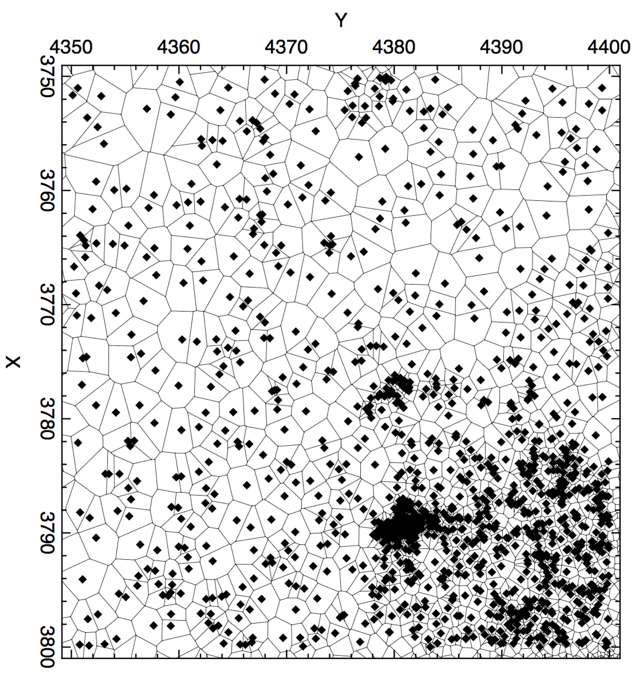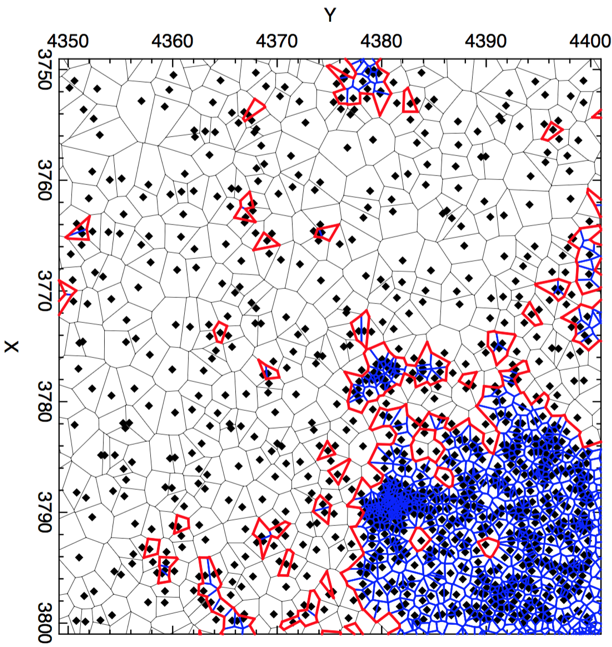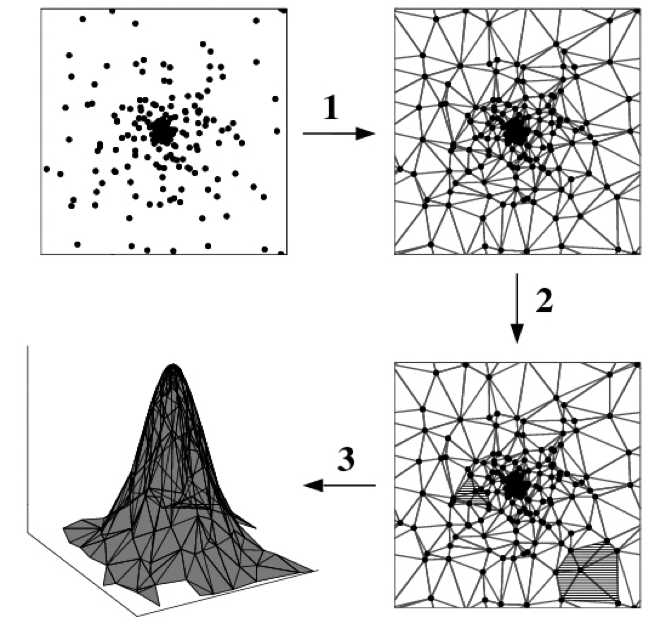## Background Maps and Source Detection with mkvtbkg

In order to efficiently identify source detection candidates, the wavdetect tool requires a background image as an input. This is because any credible source should raise above the local background level at some significance. Therefore, In CSC2, as part of the calibrate pipeline a tool is used to create background images from input evt3 and exp3 files. This tool is called mkvtbkg, and is designed to operate on single full-field observations at a given energy band, and events are processed in each CCD chip separately. In this page we provide and overview of how mkvtbkg works, and describe the output products. We also discuss how the tool is used to identify source candidates, both point-like and extended. A more detailed description of the method can be found in the memo "Constructing Background Images using Voronoi Tesselation".

## Voronoi Tessellation of a Poisson Point Process

mkvtbkg uses a Voronoi tessellation approach, based on the ideas described in Ebeling & Wiedenmann, 1993. Given a distribution of events on a chip, the Voronoi tessellation finds a unique set of non-overlapping hulls, each of which contains a single event, and that cover the entire detector region (See Fig. 1). Events near a source are closer to each other, and the resulting polygons are therefore smaller in the vicinity of a source. As a result, we can use the area of each polygon as an indication of whether that polygon is associated to a source, or to the background.

### Figure 1: Voronoi Tessellation of Events### Figure 1: Voronoi Tessellation of Events

Voronoi tessellation of a chip containing both source and background events.

## Polygon area threshold

In practice, mkvtbkg first corrects the geometric area of each polygon using the normalized effective area (from the exposure map), and then defines the dimensionless reduced area, $$\alpha$$, of each polygon as its corrected area normalized by a suitably chosen mean area. Polygons associated with sources are those whose reduced area is below a certain threshold $$\alpha_{threshold}$$, which is a free parameter of the algorithm. In CSC2, we produce polygons at different contour levels for each ObsID with extended emission by selecting different values of $$\alpha_{threshold}$$. The poly3 files contain all the contour levels for each extended source. Users interested in the detailed shape of an extended source should look at these poly3 files.

### Figure 2: Merged Polygons and Source Region### Figure 2: Merged Polygons and Source Region

Blue polygons are those with reduced areas smaller than $$\alpha_{threshold}$$. The red contours show the merged polygons. Note that they can be non-convex, and contain holes.

Polygons that are associated with sources can then be merged together to define source regions (See Fig. 2). In order to create a background image, these source regions are filtered and replaced with a uniform distribution of counts that matches the background level (see below). Note that the total fractional area of the chip associated with sources depends on the value of the mean polygon area and the threshold.

## Filtering Source Events

Next we need to filter the events contained in source polygons. It is important to realize that source polygons can be associated with either point sources and extended sources. We can therefore further partition the source polygons into small polygons ($$a < a_{large}$$) and large polygons ($$a > a_{large}$$), associated respectively with point and extended sources, such as galaxies. Events falling in small polygons are replaced with a smaller number of uniformly distributed events that match the spatial density of background events. For events in large polygons, the spatial density of replacement is chosen high enough to mask the diffuse emission, allowing wavdetect to pick up the pint sources sitting on top of this emission.

Readout streak events are filtered out by first rotating the chip so that the readout direction is aligned with the y-axis. Then a large number of uniformly distributed "events" is randomly generated and for each pixel column, we count how many of those events fall within a source polygon. If a column has a large number of its events falling on source polygons (i.e. if most of its area falls within source polygons), then the column is flagged as a readout streak column, and the polygons are flagged as readout streak polygons.

## Creating the Background Image

Once source and streak polygons have been filtered, the background image can be constructed from the resulting events by using the Delaunay triangulation field estimator. The resulting triangular tessellation has one event for each vertex of a triangle, and the local density at each vertex can be estimated as the inverse of the area of all the triangles surrounding each point (see figure). The density at all other points that are not vertices is estimated by linearly interpolating the vertex values.

### Figure 3: Delaunay Tessellation Field Estimator### Figure 3: Delaunay Tessellation Field Estimator

Schematic overview of the Delaunay tessellation field estimator steps. [https://en.wikipedia.org/wiki/Delaunay_tessellation_field_estimator#/media/File:Delaunay_tessellation_field_estimator_(overview).jpg]

The final step is to integrate the resulting density survey within each of the chip pixels. The result of the integral is the value of the background image in each pixel.

## Background images and source detection with mkvtbkg in CSC2

As pointed out earlier, mkvtbkg can be used both for creating the background image per ObsID and per chip, and also to detect regions associated with sources. In fact, mkvtbkg is the preferred method to detect sources in areas dominated by extended emission, as wavdetect tends to miss point sources sitting on top of extended emission. Because source detection is performed at the stack level for the catalog, we need to slightly adapt the method for this purpose. Therefore, in CSC2 we adopt the following procedure to detect sources in areas with extended emission.

1. For a given stack, mkvtbkg is first run for each of the observations contributing to the stack. This creates a set of observation level background images.
2. A stack-level background image is created by doing a weighted average of individual observation background images.
3. For source detection, both wavdetect and mkvtbkg are run on the stack/image. In order to decide if sources are sitting on an extended region, a comparison is performed between the number of counts $$n_{extended}$$ contained in the extended region (from mkvtbkg) and the number of counts $$n_{wavdetect}$$ contained in the wavdetect regions.
4. If $$n_{wavdetect} / n_{extended}$$ is smaller than 0.65, the point sources are considered to be sitting on an extended region, and the sources detected with mkvtbkg are recorded. For all other regions, the wavdetect detections are recorded.

The input files to mkvtbkg are:

1. An event file.
2. The energy range to perform the analysis.
3. The CCD-specific exposure map to calculate effective areas.
4. The pixel grid to perform the integration.
5. The smoothed background map to minimize edge effects during source detection.
6. A psfmap file that gives the approximate size of the PSFs at each point.

mkvtbkg produces the following output files:

1. A poly3 file containing the merged source regions at different contour levels.
2. The background image FITS file.